### Control Systems in Python - Part 3 - Root Locus Plots

In this post we can see how to make root locus plots in python. This requires the setup from part 1.

The problem is from Dorf's modern control systems AP 10.1. A three-axis pick-and-place application requires the precise movement of a robotic arm in three-dimensional space. The overshoot for a step input should be less than 13%.
a) Let Gc(s) = K, and determine the gain K that satisfies the requirement. Determine the resulting settling time (with a 2% criterion). First we compute the Routh Hurwitz table to determine the valid
range of K.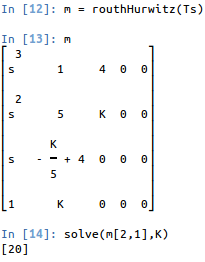We see 0<K<20 so If we let K = 2, then the step response becomes: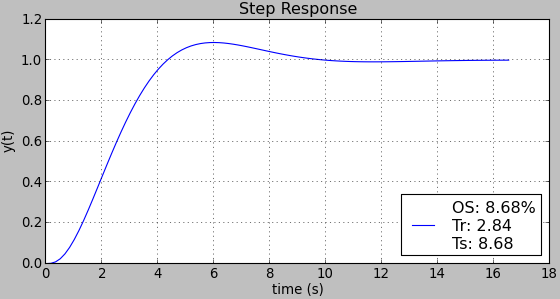which shows the settling time is 8.68 seconds and overshoot is less than 13%.

b) Use a lead network and reduce the settling time to less than 3 seconds. Since we are dealing with time response parameters the root-locus method will be used.

The new controller is: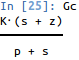the overshoot and settling time criteria lead to a damping ratio of 0.545 or more and the real part of the dominant poles must be to the left of -1.33 respectively.

If we choose our dominant poles to be at s1 = -2 +- 2j they fall in the correct region on the root locus and should get the desired response. The angle of the system L(s) at s1 is Ls 63.4o.  So letting m=0,
∠Gc(s1) =180 - 63.4 = 116.6o
the controller must add this amount. If we pick our zero to be at ½ , then using the relationship:
∠( s1+ z ) -∠(s1+p)  = 106.26o
we find p = 6.34 and K = 34.19.  This leads to the step response of: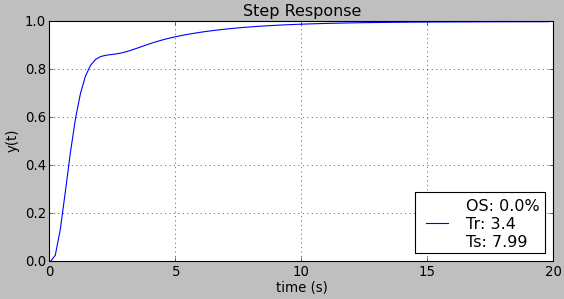from which we can see the settling time has been reduced but not enough to meet the specifications. Let's move it closer to the system pole at -1.

Reiterating through the root locus design procedure, if we move our zero out to z=¾ and recalculate p=5.86 and K=32.9, the response now is: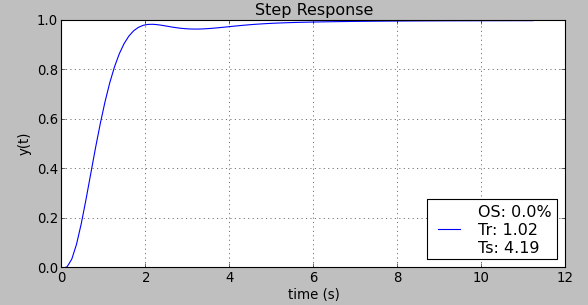which shows another good improvement in the settling time but the zero still needs moved closer to the pole at -1.

Lastly let’s set our z=0.9, then p=5.63 and K=32.5. The root locus is computed using:def rootLocus(Ts,*args,**kwargs):    num = Poly(Ts.as_numer_denom(),s).all_coeffs()    den = Poly(Ts.as_numer_denom(),s).all_coeffs()    tf = matlab.tf(map(float,num),map(float,den))    r,k = matlab.rlocus(tf,*args,**kwargs)    plt.title("Root Locus")    #plt.plot(k,r)    plt.grid()    plt.show()and the output is: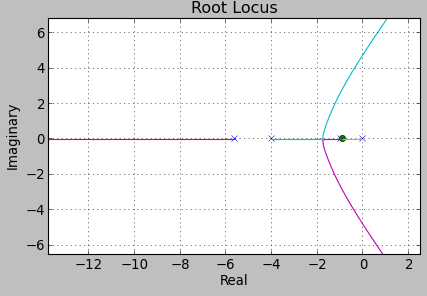resulting in the final controller of: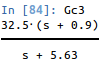who's step response is: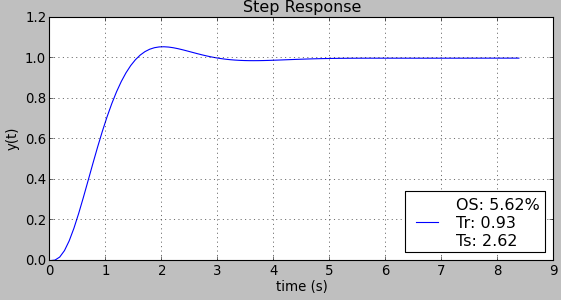which shows the controller has reduced the overshoot and settling time to within the specifications (the settling time is less than 3 seconds).

### Kivy vs React-Native for building cross platform mobile apps

I've built three apps now using Kivy and one with React-Native, just wanted to share my thoughts on both. Just a warning, I am strongly biased towards python and this is all based on opinion and experience and is thus worth what you pay for it. I don't claim to be an expert in either of these, just have worked with each for several months.  If something is incorrect I'd love to hear advice. Kivy Demo of one of the apps Pros: Nice to be able to run natively on the desktop WITHOUT a simulator Python is easy to work with Use (almost) any python library Very easy to create custom widgets Kivy properties and data binding just work. Way nicer than React's "state" / flux / redux whatever you want to call it (stupid?).  Native interfaces (pyjnius) and (pyobjc) Runs and feels pretty smooth Cons: Default widget toolkit looks like Android 4.4. Requiring you use your own widgets or a theming kit like KivyMD  if styling bothers you Creating dy

### Control Systems in Python - Part 1 - Bode and Step Response

I hate matlab with passion, yet sadly, nearly everyone uses it.  I'm a fan of Python and open source stuff so here's a simple article on how to do some common control systems stuff in Python. First we need to make sure the environment is setup. Install IPython (or you can use any other python shell, but a unicode supported shell is preferred) Install python-control (numpy, scipy) Install sympy These should do if your on Ubuntu/debian: sudo apt - get install python - sympy python-numpy python-scipy python-matplotlib ipython Then you need to install python control, see How to download and install python-control Intro to using Sympy Open ipython and run the following: import sympy from sympy import * sympy.init_printing() s = Symbol('s') Now we can do things like define transfer functions using the symbolic variable s. We can expand the bottom using the .simplify() method and we can do something more complex like... which is really nice because it

### Control Systems in Python - Part 2 - Routh Hurwitz

In my last post Control Systems in Python Part 1 , i described how to setup and use Python for doing some basic plotting of transfer functions. One of the biggest benefits of using sympy vs numeric packages like matlab/numpy/scipy is the fact that you can use symbolic variables. This post includes a function for computing the Routh Hurwitz table (Note: It does not work for row's of zeros). Lets do my control systems design homework problem together :) (Warning: I have not verified if this answer is right so please correct me if it’s not!) The Problem The problem is DP 9.11 from Dorf & Bishop’s Modern Control Systems. ISBN 0136024580. Basically we have to design a controller to compensate for a system with a time delay. The controller is: And the system is: First we approximate the exponential term with a 2nd order polynomial using pade(0.4,2) such that: Thus the approximated system is: Using frequency response methods, design the controller so that th